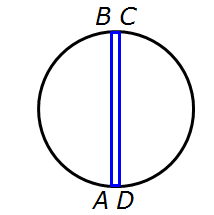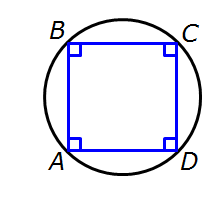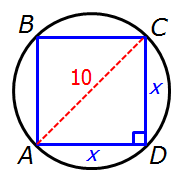It is currently 26 Sep 2020, 00:30### GMAT Club Daily Prep

#### Thank you for using the timer - this advanced tool can estimate your performance and suggest more practice questions. We have subscribed you to Daily Prep Questions via email.

Customized
for You

we will pick new questions that match your level based on your Timer History

Track

every week, we’ll send you an estimated GMAT score based on your performance

Practice
Pays

we will pick new questions that match your level based on your Timer History

#### Not interested in getting valuable practice questions and articles delivered to your email? No problem, unsubscribe here.# In the ﬁgure above, the diameter of the circle is 10.Question banks Downloads My Bookmarks Reviews Important topics
Author Message
TAGS:
FounderJoined: 18 Apr 2015
Posts: 13362
Followers: 289

Kudos [?]: 3396 , given: 12202

In the ﬁgure above, the diameter of the circle is 10. [#permalink]
Expert's post00:00

Question Stats:72% (00:43) correct27% (01:15) wrongbased on 113 sessions
Attachment:#GREpracticequestion The area of quadrilateralABCD.jpg [ 9.19 KiB | Viewed 5182 times ]

In the figure above, the diameter of the circle is 10.

 Quantity A Quantity B The area of quadrilateral ABCD 40

A)The quantity in Column A is greater.
B)The quantity in Column B is greater.
C)The two quantities are equal.
D)The relationship cannot be determined from the information given.

Practice Questions
Question: 3
Page: 151
Difficulty: medium
[Reveal] Spoiler: OA

_________________

Need Practice? 20 Free GRE Quant Tests available for free with 20 Kudos
GRE Prep Club Members of the Month: Each member of the month will get three months free access of GRE Prep Club tests.

FounderJoined: 18 Apr 2015
Posts: 13362
Followers: 289

Kudos [?]: 3396 , given: 12202

Re: In the ﬁgure above, the diameter of the circle is 10. [#permalink]
Expert's post
Solution

The best way to solve this question is its evaluation upfront..

More often than not people start to calculate the arcs and then make the difference between the circle area and the triangle area.

Instead if you figure out that the figure DO NOT drwan the scale and that could be in this way$$OR$$You do not have inough information to come up with a solution

So the answer is $$D$$
_________________

Need Practice? 20 Free GRE Quant Tests available for free with 20 Kudos
GRE Prep Club Members of the Month: Each member of the month will get three months free access of GRE Prep Club tests.

DirectorJoined: 03 Sep 2017
Posts: 518
Followers: 2

Kudos [?]: 432 , given: 66

Re: In the ﬁgure above, the diameter of the circle is 10. [#permalink]
Actually, the source states also that the diameter of the circle is 10. I think it makes it a little bit trickier
VPJoined: 20 Apr 2016
Posts: 1302
WE: Engineering (Energy and Utilities)
Followers: 22

Kudos [?]: 1312 , given: 251

Re: In the ﬁgure above, the diameter of the circle is 10. [#permalink]
IlCreatore wrote:
Actually, the source states also that the diameter of the circle is 10. I think it makes it a little bit trickier

Question corrected as per source.
_________________

If you found this post useful, please let me know by pressing the Kudos Button

Rules for Posting

Got 20 Kudos? You can get Free GRE Prep Club Tests

GRE Prep Club Members of the Month:TOP 10 members of the month with highest kudos receive access to 3 months GRE Prep Club testsGRE InstructorJoined: 10 Apr 2015
Posts: 3835
Followers: 148

Kudos [?]: 4491  , given: 69

Re: In the ﬁgure above, the diameter of the circle is 10. [#permalink]
2
KUDOS
Expert's post
Carcass wrote:
Attachment:

In the figure above, the diameter of the circle is 10.

 Quantity A Quantity B The area of quadrilateral ABCD 40

A)The quantity in Column A is greater.
B)The quantity in Column B is greater.
C)The two quantities are equal.
D)The relationship cannot be determined from the information given.

Practice Questions
Question: 3
Page: 151
Difficulty: medium

First of all, it's important not to read too much into the diagram.
All we can glean from the diagram is that we have a quadrilateral that is inscribed in the circle.
That's it!

So, first recognize that the inscribed quadrilateral COULD be a very very very narrow rectangle like this.Notice that this quadrilateral COULD be so thin that its area is very very close to zero.
So, for this particular quadrilateral we get:
Quantity A: a very very small area that's close to zero
Quantity B: 40
In this case, Quantity B is greater.

Alternatively, we COULD make the quadrilateral quite large.
In fact, we could make it a SQUARE.So, if the inscribed quadrilateral is a square, what is its area?
To find out, let's draw a diagonal.One of our circle properties tells us that this diagonal must be the diameter of the circle, which we know is 10
To find the area of the square, we need to know the length of each side.
So, let's let x = the length of each side.

Since ACD is a RIGHT TRIANGLE, we can apply the Pythagorean Theorem to get: x² + x² = 10²
Simplify to get 2x² = 100
Divide both sides by 2 to get: x² = 50

This means the area of the square = 50
We know this because the area of the square = (x)(x) = x², and we just learned that x² = 50

So, for this particular quadrilateral we get:
Quantity A: 50
Quantity B: 40
In this case, Quantity A is greater.

RELATED VIDEO

_________________

Brent Hanneson – Creator of greenlighttestprep.com
If you enjoy my solutions, you'll like my GRE prep course.Senior ManagerJoined: 10 Feb 2020
Posts: 482
Followers: 1

Kudos [?]: 117 , given: 293

Re: In the ﬁgure above, the diameter of the circle is 10. [#permalink]
GreenlightTestPrep wrote:
Carcass wrote:
Attachment:

In the figure above, the diameter of the circle is 10.

 Quantity A Quantity B The area of quadrilateral ABCD 40

A)The quantity in Column A is greater.
B)The quantity in Column B is greater.
C)The two quantities are equal.
D)The relationship cannot be determined from the information given.

Practice Questions
Question: 3
Page: 151
Difficulty: medium

First of all, it's important not to read too much into the diagram.
All we can glean from the diagram is that we have a quadrilateral that is inscribed in the circle.
That's it!

So, first recognize that the inscribed quadrilateral COULD be a very very very narrow rectangle like this.Notice that this quadrilateral COULD be so thin that its area is very very close to zero.
So, for this particular quadrilateral we get:
Quantity A: a very very small area that's close to zero
Quantity B: 40
In this case, Quantity B is greater.

Alternatively, we COULD make the quadrilateral quite large.
In fact, we could make it a SQUARE.So, if the inscribed quadrilateral is a square, what is its area?
To find out, let's draw a diagonal.One of our circle properties tells us that this diagonal must be the diameter of the circle, which we know is 10
To find the area of the square, we need to know the length of each side.
So, let's let x = the length of each side.

Since ACD is a RIGHT TRIANGLE, we can apply the Pythagorean Theorem to get: x² + x² = 10²
Simplify to get 2x² = 100
Divide both sides by 2 to get: x² = 50

This means the area of the square = 50
We know this because the area of the square = (x)(x) = x², and we just learned that x² = 50

So, for this particular quadrilateral we get:
Quantity A: 50
Quantity B: 40
In this case, Quantity A is greater.

RELATED VIDEO

why do we need to change the shape? cant we calculate the values as per the given diagram?
_________________

Ever Tried? Ever Failed? No Matter. Try Again. Fail Again. Fail Better!!GRE InstructorJoined: 10 Apr 2015
Posts: 3835
Followers: 148

Kudos [?]: 4491  , given: 69

Re: In the ﬁgure above, the diameter of the circle is 10. [#permalink]
2
KUDOS
Expert's post
Farina wrote:
why do we need to change the shape? cant we calculate the values as per the given diagram?

What values are you referring to?
All we're told is that the diameter of the circle is 10.
Other than the fact that the quadrilateral is inscribed in the circle, there's no other information that will "lock in" the area of the quadrilateral.
As such, we need to consider various possible scenarios.

Cheers,
Brent
_________________

Brent Hanneson – Creator of greenlighttestprep.com
If you enjoy my solutions, you'll like my GRE prep course.Re: In the ﬁgure above, the diameter of the circle is 10.   [#permalink] 27 Apr 2020, 13:30
Display posts from previous: Sort by

# In the ﬁgure above, the diameter of the circle is 10.Question banks Downloads My Bookmarks Reviews Important topicsPowered by phpBB © phpBB Group Kindly note that the GRE® test is a registered trademark of the Educational Testing Service®, and this site has neither been reviewed nor endorsed by ETS®.